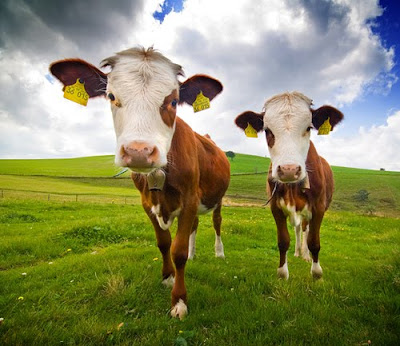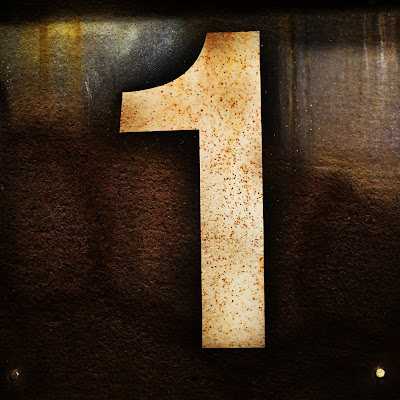## Wednesday, 2 June 2010

### 2 (Two)

2 (two) is a number, numeral, & glyph.
It is the natural number following 1 and preceding 3.
An integer is called even if it is divisible by 2.
Two is the smallest and the first prime number.
Two is also the only even prime number - sometimes called "the oddest prime".Two Cows
2 - Birds - Cow - Glyph - Integer - Numeral - Prime Number - Two

## Sunday, 23 May 2010

### 1 (One)Image by horizontal.integration (cc) (flickr)

"1 (one) is a number, numeral, and the name of the glyph representing that number. It represents a single entity, the unit of counting or measurement. For example, a line segment of "unit length" is a line segment of length 1." (Wikipedia)

### Visuopedia Numbers - A Visual Dictionary Encyclopedia About Numbers

Visuopedia Numbers is a Visual Dictionary Encyclopedia about numbers.

This blog will be devoted to the visual representation of numbers.